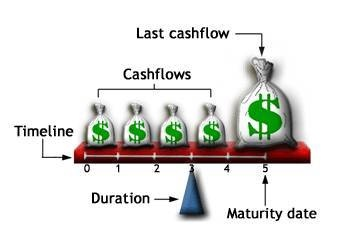# 千橙工坊

## 当我们谈论"久期"(Macaulay Duration)的时候, 在说什么?

2018-05-21

-- 周召(via. 知乎) 这样形象描述.$$\begin{equation} D = \frac{\sum_{t=1}^T \frac{c_t}{(1+y)^t}\times t}{P} \text {, 麦考利久期的計算公式} \end{equation}$$

$$MacD = \frac{\sum_{t=1}^T PV\left( c_t\right) \times t}{P} = \sum_{t=1}^T \left[ \frac{PV\left( C_t\right)}{P} \times t \right] \tag{2}$$

• MacD是久期，
• P是債券當前的市場價格，
• $PV\left( c_t\right)$ 是債券未來第t期可現金流（利息或資本）的現值，
• T是債券的到期時間。
• t為從當前到t時刻現金流發生的持續時間。
• y為債券的風險程度相適應的收益率。假設未來所有現金流的貼現率(discount ratio)都固定為y。可以理解为IRR 或者 YTM 或者 MWR.

### 定理

1. 零息债券(Zero-coupon bonds)的久期等于它的到期时间
2. 直接债券(Direct bond)的久期小于或等于它的到期时间
3. 统一公债(Unified Bond)的久期等于$1+ {1\over Y}$，其中Y是计算现值的贴现率
4. 到期时间相同的条件下，息票率(Coupon rate)越高，久期越短
5. 息票率不变的条件下，到期时间越久，久期也越长
6. 其他条件不变的情况下，债券的到期收益率(YTM)越低，久期越长

### 債券凸性與馬考勒久期之間的關係

1. 久期的维基百科: http://wiki.mbalib.com/zh-tw/%E4%B9%85%E6%9C%9F

CommentsWrite a Comment